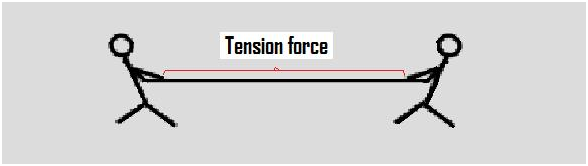# Tension ForceThe tension force is defined as the force that is transmitted through a rope, string or wire when pulled by forces acting from opposite sides. The tension force is directed over the length of the wire and pulls energy equally on the bodies at the ends.

Every physical object which is in contact exerts some force on one another. These contact forces are assigned with names based on kind of objects. If one of the force exerting object is a rope, cable or chain, you can call it as tension.

Cables and Ropes can be used for exerting forces since they can transfer a force over a specific distance efficiently (e.g. the rope length). Please note that tension is the pulling force since ropes cannot push effectively. Pushing with a rope causes the rope to go slack and lose the tension that allowed it to pull in the original place. This might seem obvious but when it is about drawing the forces acting on an object, some often draw the force of tension going towards the wrong direction. Hence, tension can only pull an object.

You will be presented with massless ropes and cables in almost every situation in classical mechanics. When a rope is massless, it transfers the force from one end to other perfectly.

For instance, if a person pulls a massless rope with a force of 20 N, the block also experiences a force of 20 N. All massless ropes experience two opposite and equal tension forces. Here, the person pulling a block with a rope, the rope experiences a net force. Therefore, all massless ropes experience two opposite and equal tension forces. In case of a person pulling a block, the rope experiences a tension towards one direction from the pull and the tension in another direction from the reactive force of the block.

## Tension Force Formula

The tension on a body can be expressed numerically as:

T = mg + ma

Where;

T indicates tension, N

m indicates mass, kg

g indicates gravitational force, 9.8 m/s2

A indicates acceleration, m/s2#### 1 Comment

1. Anonymous

This info helped so much and I got though my exam